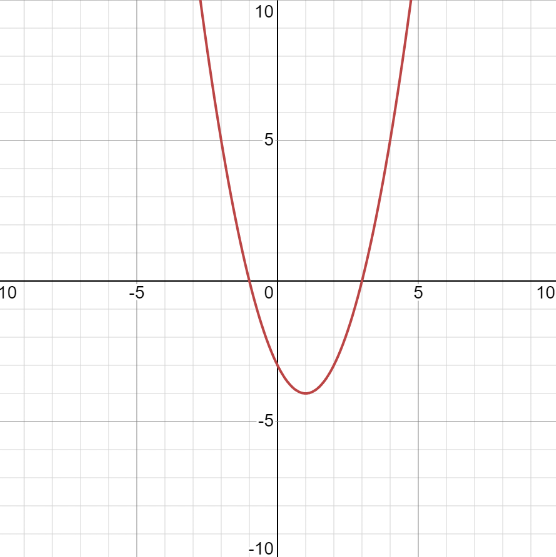# If f(x)=x^2-2x+3 how do you find f(a+h)-f(a)/h?osnomu3 2021-12-24 Answered
If $f\left(x\right)={x}^{2}-2x+3$ how do you find f(a+h)-f(a)/h?
You can still ask an expert for help

• Questions are typically answered in as fast as 30 minutes

Solve your problem for the price of one coffee

• Math expert for every subject
• Pay only if we can solve itdeginasiba
Substitute $a+h$ and $a$ for $x$ in the formula for f(x) and simplify to find:
$\frac{f\left(a+h\right)-f\left(a\right)}{h}=2a-2+h$
$f\left(x\right)={x}^{2}-2x+3$
Then: $\frac{f\left(a+h\right)-f\left(a\right)}{h}$
$\frac{{a}^{2}+2ah+{h}^{2}-2a-2h+3-{a}^{2}+2a-3}{h}$
$=-2a-2+h$
So:
$\underset{h\to 0}{lim}\frac{f\left(a+h\right)-f\left(a\right)}{h}=\underset{h\to 0}{lim}=\left(2a-2+h\right)=2a-2$
This is the derivative of $f\left(x\right)$ at $x=a$
###### Not exactly what you’re looking for?Timothy Wolff

I have plotted it for you!###### Not exactly what you’re looking for?user_27qwe